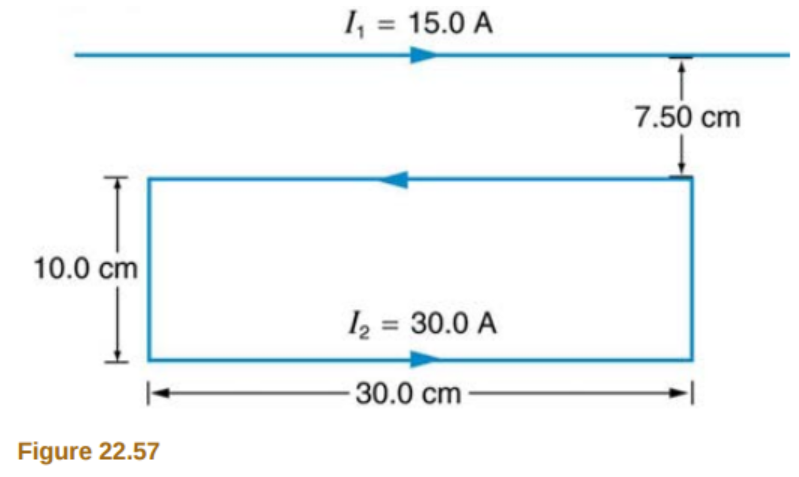# Problem: Figure 22.57 shows a long straight wire near a rectangular current loop. What is the direction and magnitude of the total force on the loop?

###### FREE Expert Solution

Force per unit length is given by:

$\overline{)\frac{\mathbf{F}}{\mathbf{L}}{\mathbf{=}}\frac{{\mathbf{\mu }}_{\mathbf{0}}{\mathbf{i}}_{\mathbf{1}}{\mathbf{i}}_{\mathbf{2}}}{\mathbf{2}\mathbf{\pi r}}}$

Force on the top side of the loop:

r = 7.5 cm(1m/100cm) = 0.075 m

Length of the straight conductor, L = 30 cm(1m/100cm) = 0.30 m

i1 = 15 A

i2 = 30 A

92% (50 ratings)###### Problem Details

Figure 22.57 shows a long straight wire near a rectangular current loop. What is the direction and magnitude of the total force on the loop?